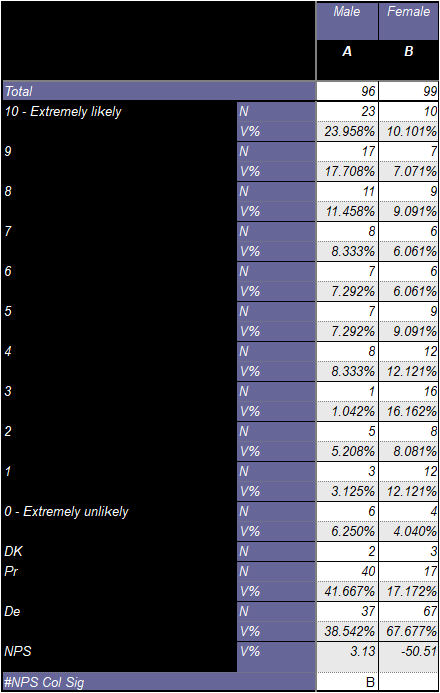Scroll

# Programming Col Sig (NPS): Classical Student Test

Follow
 Summary This article demonstrates how to script the Student Test formula for testing Net Promoter Scores (NPS) on 2 independent populations. The set-up allows you to use regular in-built col sig on the responses of the question and switch to the bespoke NPS col sig calculation when it gets to the NPS row in the table. Applies to Askia Analyse. Written for Researchers, Developers, Data Processors, Statisticians. Keywords Table arithmetic, Forloops, Coordinates, Data, Calculation arithmetic, Normal distribution, Bespoke calculation, Filtered, Student Test, Sigma, T-test, Confidence interval, 95%, 90%, 99%, FilterBy, GetColSigLetter, Two-tailed test, NPS, Formula, Net Promoter.

The .qes file and portfolio containing the examples discussed is attached: NPS-Col-Sig.zip.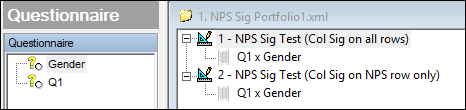Open the first tab definition of the portfolio: NPS Sig Test (Col Sig on all rows). We have a regular NPS set-up like this: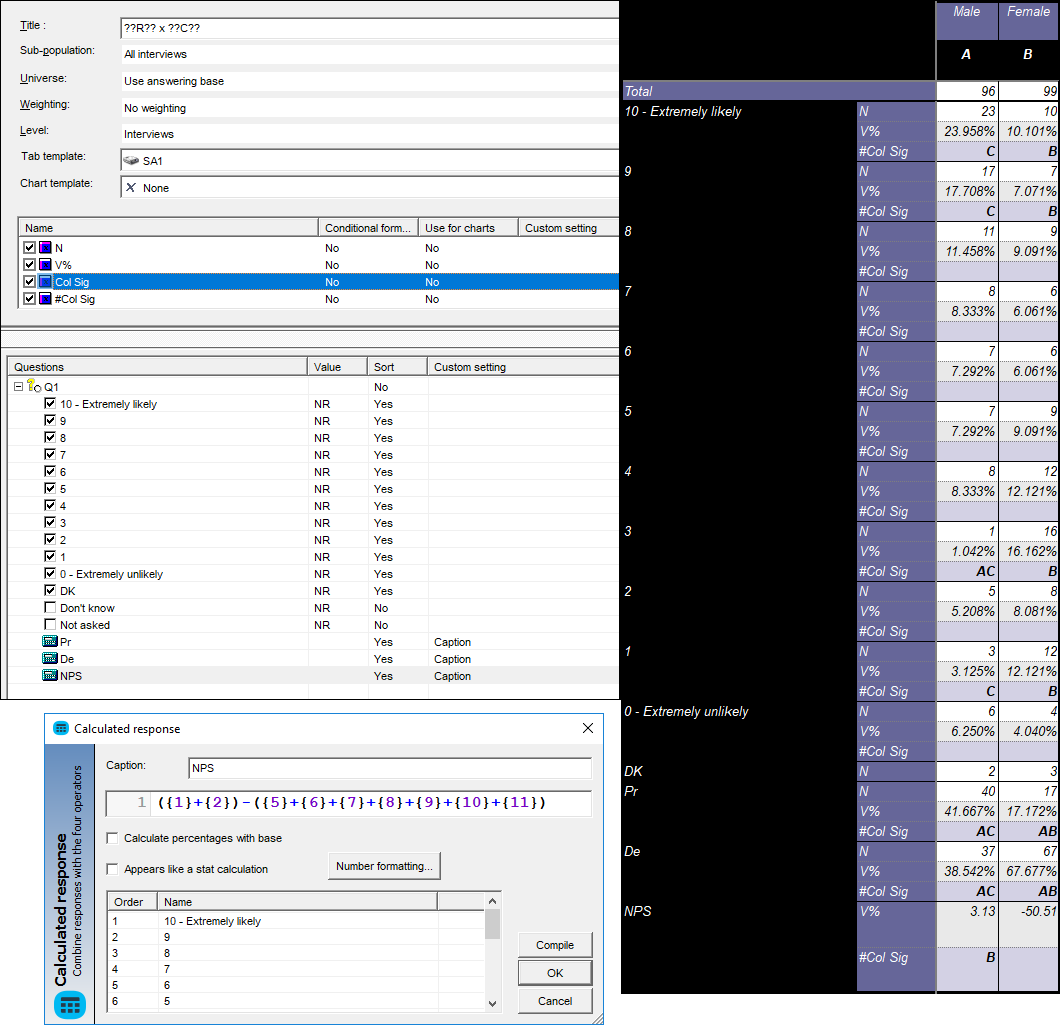For every row apart from the NPS we want to show the inbuilt classical student col sig test:For the NPS row we want to use the standard student test formula for significant difference between 2 Net Promoter Scores in 2 independent populations.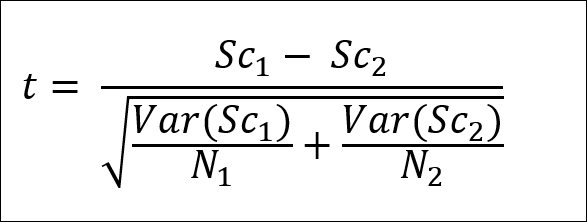Where:

t is the sigma value

• If t > 1.645, significance at 90%
• If t > 1.960, significance at 95%
• If t > 2.576, significance at 99%

Sc is the NPS for each independent population

N is the base size in the NPS row for each independent population

Var(Sc) is the variance of the NPS:

• Var(Sc) = Abs(Pr + De – (Pr – de)²)

• Pr is the vertical % of promoters
• De is the vertical % of detractors
• Abs is the absolute value i.e. if the contents of the brackets is negative, we make this positive

The syntax:

```Dim numVisibleCalcsNPS = 2 ' number of visible calculations in your NPS response (assumes #ColSig is last calculation)
Dim numSetPrY = 0
Dim numSetDeY = 0
Dim numSetNPSY = 0

Dim j
Dim k
For j = CurrentTable.StartY To CurrentTable.MaxY
For k = 1 to CurrentTable.StartX ' scan all columns until start of data for "Pr", "De" and "NPS" response captions
If InStr( "Pr", CurrentTable.GetCell(k,j).Text) > 0 Then
numSetPrY = j ' find y-coord of "Pr" label
Endif

If InStr( "De", CurrentTable.GetCell(k,j).Text) > 0 Then
numSetDeY = j ' find y-coord of "De" label
Endif

If InStr( "NPS", CurrentTable.GetCell(k,j).Text) > 0 Then
numSetNPSY = j ' find y-coord of "NPS" label
Endif
Next k
Next j

Dim numPr1 = CurrentTable.GetCell(CurrentCell.X, numSetPrY + 1).Value ' use y-coord of "Pr" label (+1 to get to % row)
Dim numDe1 = CurrentTable.GetCell(CurrentCell.X, numSetDeY + 1).Value ' use y-coord of "De" label (+1 to get to % row)

Dim numCoVar1 = (numPr1 + numDe1) - (numPr1 - numDe1).Pow(2)
Dim numNPSc1 = numPr1 - numDe1
Dim dN1 = CurrentCell.Row.Question.Data.FilterByX().Size()
Dim StrColSig = ""

Dim dSigma = 0
Dim dDenom = 0

Dim i
For i = CurrentTable.StartX To CurrentTable.MaxX
Dim numPr2 = CurrentTable.GetCell(i, numSetPrY + 1).Value ' use y-coord of "Pr" label (+1 to get to % row)
Dim numDe2 = CurrentTable.GetCell(i, numSetDeY + 1).Value ' use y-coord of "De" label (+1 to get to % row)
Dim numCoVar2 = (numPr2 + numDe2) - (numPr2 - numDe2).Pow(2)
Dim numNPSc2 = numPr2 - numDe2
Dim dN2 = CurrentCell.Row.Question.Data.FilterByX(i).Size()

dDenom = ((numCoVar1/dN1) + (numCoVar2/dN2)).Abs().Pow(0.5)

dSigma = (numNPSc1 - numNPSc2) / dDenom

If dSigma.Abs() > 1.96 and numNPSc1 > numNPSc2 Then ' test at 95% and only show colsig letter in the column with highest value
StrColSig = StrColSig + CurrentTable.GetColSigLetter(i,2)
Endif

Next i

If CurrentCell.Y <> numSetNPSY + (numVisibleCalcsNPS - 1) Then ' for all rows apart from the NPS row, return the (hidden) inbuilt col sig calc  Return CalcStr(3)Else  Return StrColSig ' otherwise return the col sig programmed aboveEndif ```

Some key points to note about the syntax:

• The positions of the values: Pr% (promoters), De% (detractors) and NPS are determined by scanning the columns up to the data start (columns 1 to CurrentTable.StartX) for the text "Pr", "De" and "NPS" respectively. If your tables have different captions then you need to change these parts of the syntax (lines 10, 14 & 18).
• In the first line you have a parameter numVisibleCalcsNPS you can change. It's the number of visible calculations for your NPS response. The default is two as we assume you just want to show the NPS and the col sig for it: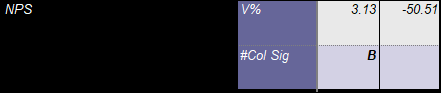• The col sig letter is only shown in the column with the highest NPS value. If you want to show it in both you can change (If dSigma.Abs() > 1.96 and numNPSc1 > numNPSc2 Then) to be (If dSigma.Abs() > 1.96 Then). It may be useful to check here for the properties of the GetColSigLetter method.

What if you want to show the col sig for the NPS only and none of the other rows in your table?

Well there are some simple steps to achieve this:

• Untick the inbuilt 'Col sig' calculation you have in your tab template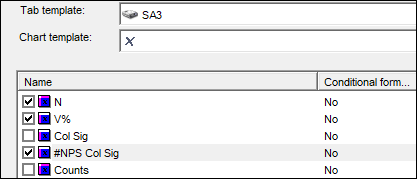• Go into the Properties > Script of the '#Col sig' calculation
• Tick 'Appears like a stat calculation'
• Comment out 4 of the last 5 lines in the syntax to leave only 'Return StrColSig'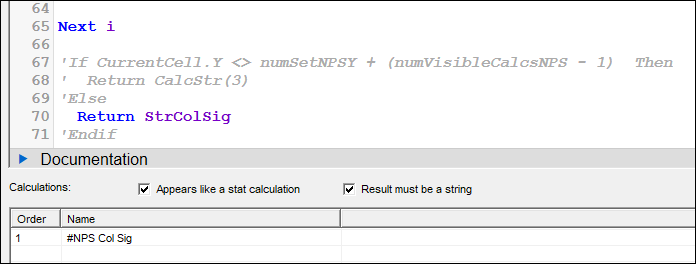• Save and run the results